# Asap 1. The bracket is made of steel (Young's modulus 200 GPa; Poisson's ratio 0.3). When the force P is applied to the bracket, the gages in the strain rosette at point A have the followi...

Asap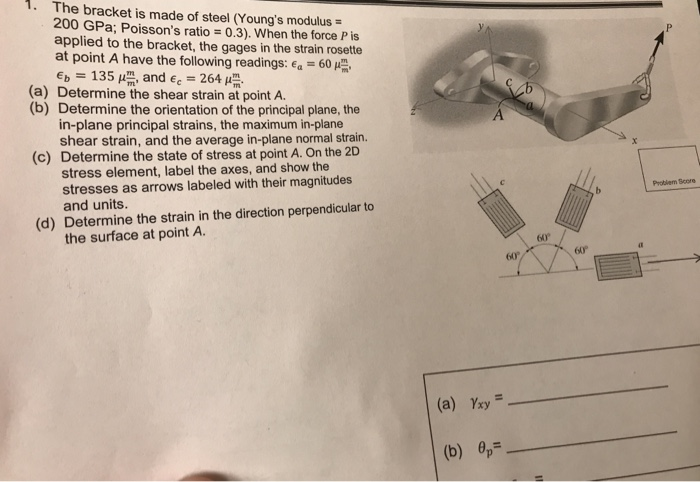1. The bracket is made of steel (Young's modulus 200 GPa; Poisson's ratio 0.3). When the force P is applied to the bracket, the gages in the strain rosette at point A have the following readings: E.-60 μ . Ep 135 μ l, and E.-264 μ (a) Determine the shear strain at point A. (b) Determine the orientation of the principal plane, the in-plane principal strains, the maximum in-plane shear strain, and the average in-plane normal strain. Determine the state of stress at point A. On the 2D stress element, label the axes, and show the stresses as arrows labeled with their magnitudes and units. (c) Problem Score (d) Determine the strain in the direction perpendicular to the surface at point A. 60 60 60 (a) Yxy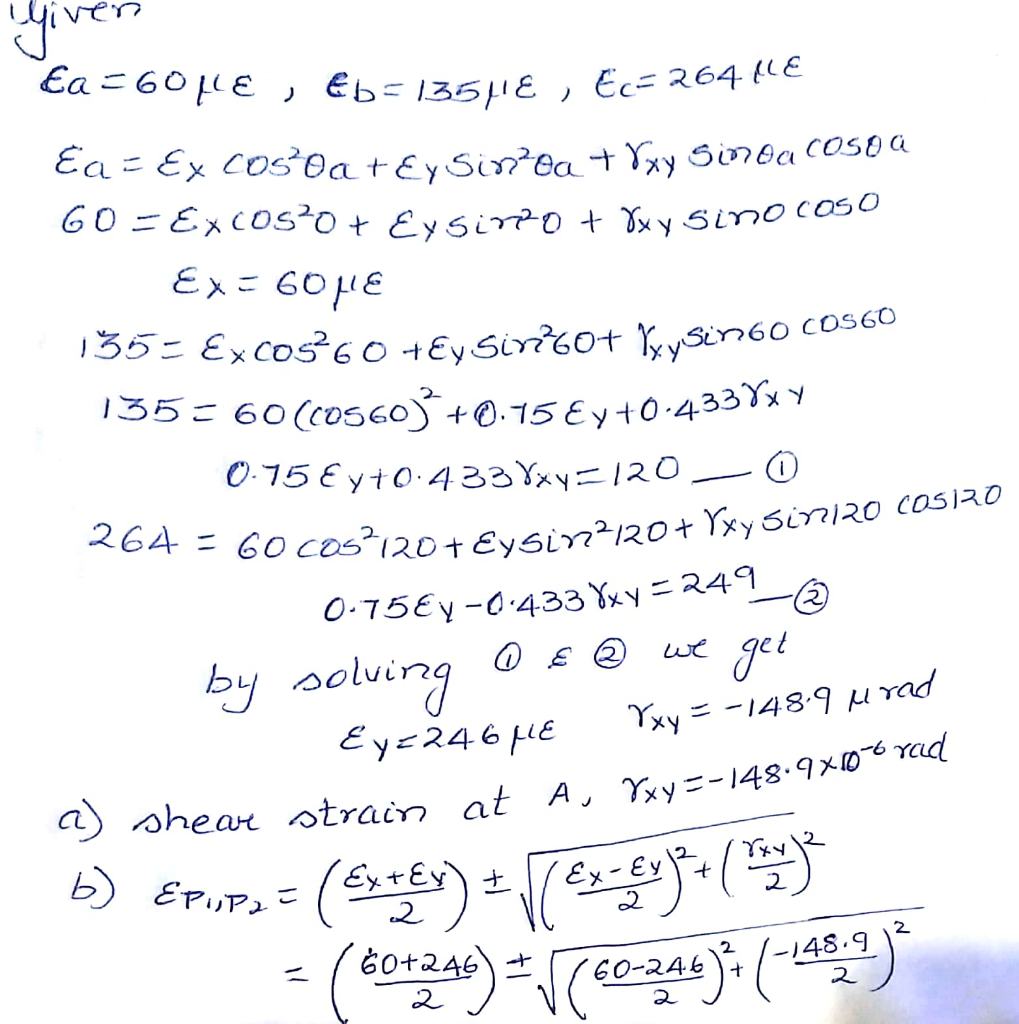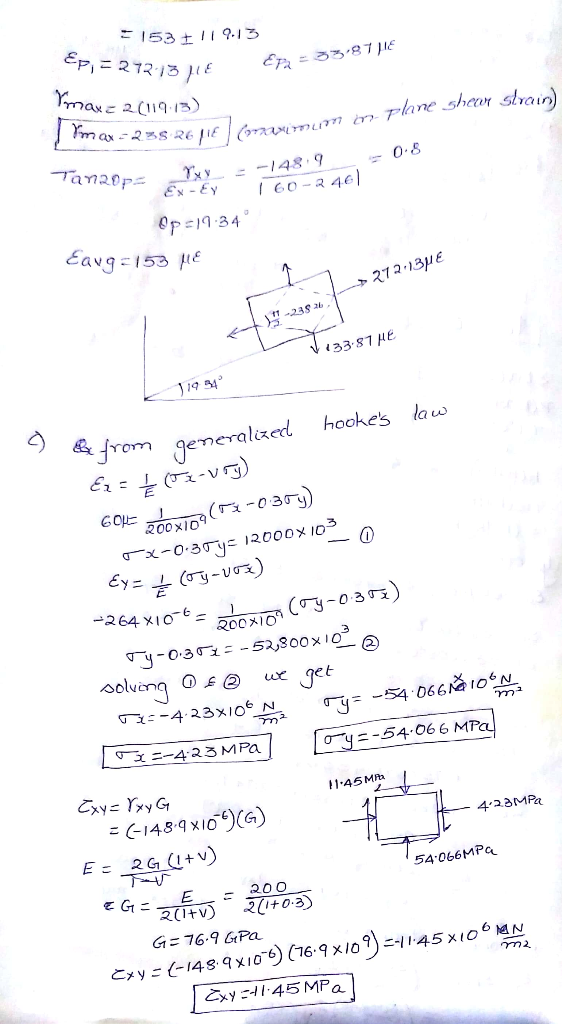#### Earn Coin

Coins can be redeemed for fabulous gifts.

Similar Homework Help Questions
• ### a cylindrical tank is made from A-36 OBLEM 2: The cylindrical tank is made from A-36 steel with a yield strength ayie 250 MPa, modulus ef elasticity &-200 GPa and Poisson's ratio v 0.3. W...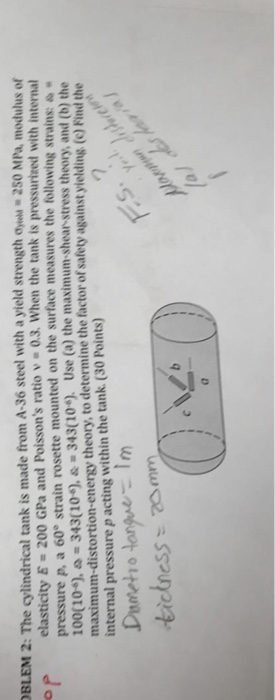a cylindrical tank is made from A-36 OBLEM 2: The cylindrical tank is made from A-36 steel with a yield strength ayie 250 MPa, modulus ef elasticity &-200 GPa and Poisson's ratio v 0.3. When the tank is pressuri pressure p, a 60 strain rosette mounted on the surface measures the following strains: & 100(10), 343(10), maximum-distortion-energy theory, to determine the factor of safety against yielding (c) Find the internal pressure p acting within the tank. (30 Points) 343(10) Use...

• ### Question 2 I (a) Using a 459 strain gauge rosette placed on a mild steel structure,...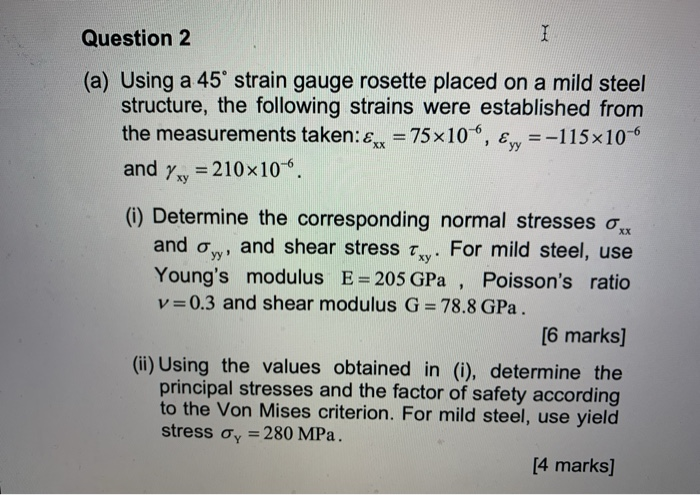Question 2 I (a) Using a 459 strain gauge rosette placed on a mild steel structure, the following strains were established from the measurements taken:£x = 75x10", Eyy =-115x10-6 = 210 10-6 and Yxy yy (i) Determine the corresponding normal stresses Ox and and shear stress txy. For mild steel, use Young's modulus E= 205 GPa , Poisson's ratio v=0.3 and shear modulus G = 78.8 GPa. [6 marks] (ii) Using the values obtained in (i), determine the principal stresses...

• ### PROBLEM 1: The 45° strain rosette is mounted on the link of the backhoe. The following...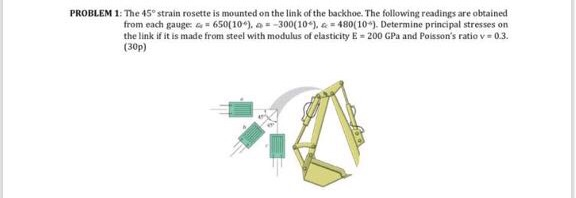PROBLEM 1: The 45° strain rosette is mounted on the link of the backhoe. The following readings are obtained from each gauge 650[10). = -300(10) = 480(10"). Determine principal stresses on the link if it is made from steel with modulus of elasticity E - 200 GPa and Poisson's ratio v 0.3. (30p) A 2 Strain Rosettes Transformation equations (45°configuration) Ex = {a Ey = {c Yxy = 2ęp – (@a+ ac) Transformation equations (60°configuration) Ex = {a Ey =...

• ### Example: 1.2 A wrench is made of steel (E=210 GPa, v=0.3) with elastic constants of strain...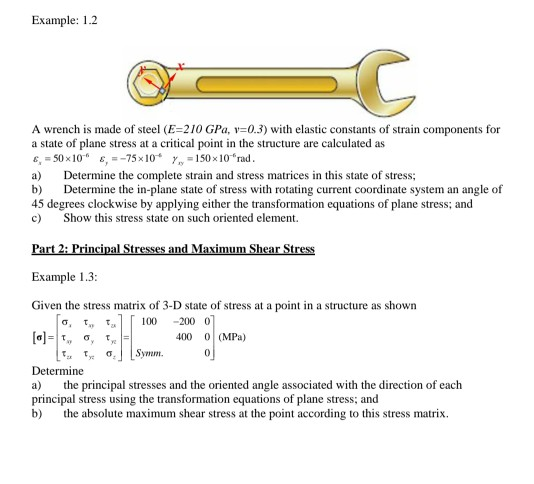Example: 1.2 A wrench is made of steel (E=210 GPa, v=0.3) with elastic constants of strain components for a state of plane stress at a critical point in the structure are calculated as 6, 50 x 10 = -75x10* 1 = 150 x 10"rad. a) Determine the complete strain and stress matrices in this state of stress, b) Determine the in-plane state of stress with rotating current coordinate system an angle of 45 degrees clockwise by applying either the transformation...

• ### Question 1 An end capped cylindrical pressure vessel with internal pressure p = fully supported at...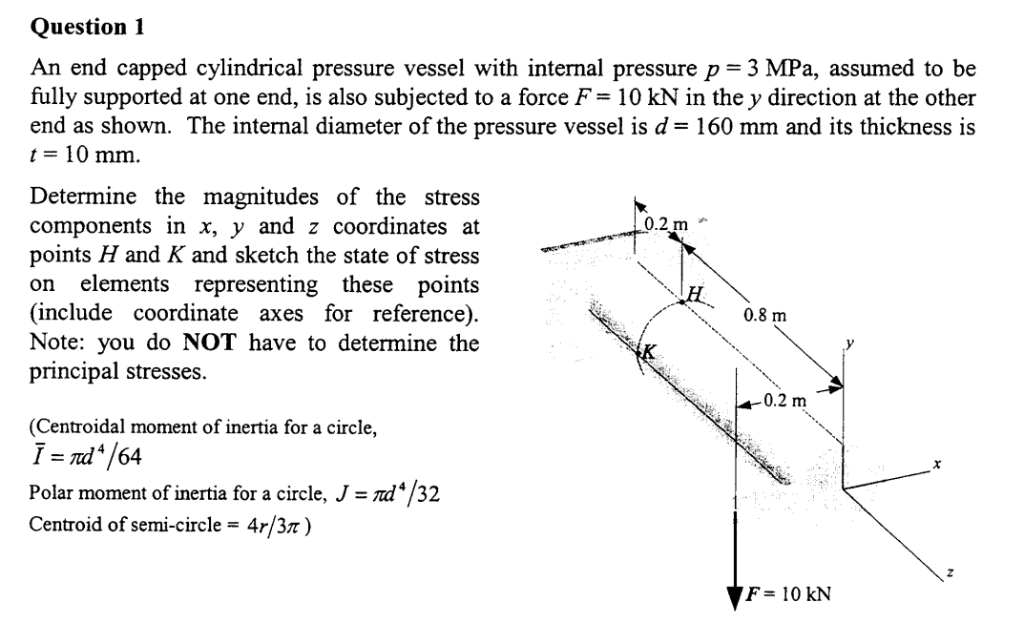Question 1 An end capped cylindrical pressure vessel with internal pressure p = fully supported at one end, is also subjected to a force F= 10 kN in the y direction at the other end as shown. The internal diameter of the pressure vessel is d= 160 mm and its thickness is t= 10 mm 3 MPa, assumed to be Determine the magnitudes of the stress components in x, y and z coordinates at points H and K and sketch...